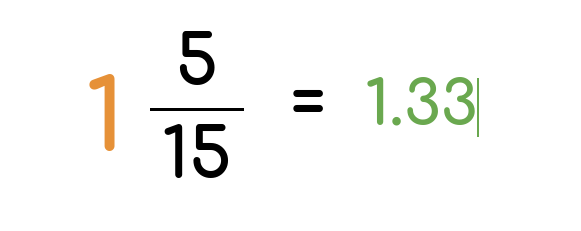# Mixed Number to Decimal Calculator

## How to quickly turn mixed numbers into decimalsDecimals offer a clearer indication of estimation than mixed numbers. Thus, converting them to decimals will simplify and expedite your solving process.

That said, here at Calcopolis, we'll provide you with the right tools to deal with everything that involves math! Our mixed number-to-a-decimal calculator is an excellent tool you can use.

## How Does the Calculator Work?

The calculator accurately finds the decimal equivalent of fractions and mixed numbers as quickly as possible.

Not only will our software convert integers and fractions to decimals, but it will also walk you through the entire process (talk about handy!)

The interface of this converter is user-friendly. So, even if you're not that familiar with smartphones or computers, you can still easily navigate and use them.

## How to Convert Mixed Number Into Decimal

Converting mixed numbers or fractions into decimals is simple and easy. Have a look at the methods our calculator uses.

### Method 1: Fraction to Decimal

Mixed numbers are basically just whole numbers with fractions. When attempting to convert them into their decimal equivalents, there are two basic steps to remember.

The first step is to convert your fraction to decimal form. You can do this by dividing the fraction's numerator by its denominator.

Once you have the decimal equivalent of your fraction, add the whole number part to it to get the decimal value of your mixed number.

This method works even if the fraction on your mixed number is an improper fraction.

#### Example:

Consider this mixed number: 8 1/5.

1. First, convert the fraction into its decimal form. The fraction is 1/5, so you get 1 ÷ 5
2. 1 ÷ 5 equals 0.2
3. Add 0.2 to the whole number. You get 8 + 0.2
4. 8 + 0.2 equals 8.2
5. The decimal equivalent of 8 1/5 is 8.2

Aside from converting the fraction to decimal, you can also use fraction addition to find the decimal equivalent of your mixed number.

To begin, separate the whole number and the fraction part of the mixed number into two fractions. Convert your whole number into an improper fraction with 1 as the denominator.

You must add your fractions once you have obtained them. But first, you must determine the lowest common multiple (LCM) of both denominators.

After you've calculated the LCM, multiply the numerator and denominator of the first fraction by the LCM. After that, you can start adding the two fractions.

Add the numerator of both fractions, but keep the denominator the same. With the resulting fraction, you will get its decimal equivalent by dividing the numerator by its denominator.

#### Example:

Consider this mixed number: 5 1/5.

1. Convert the mixed number into two separate fractions: 5/1 and 1/5
2. Find the LCM of both denominators: LCM is 5
3. Multiply 5/1 by 5 before adding: (5/1 x 5/5) + 1/5
4. Add the numerators to get 25/5 + 1/5 = 26/5
5. Divide the resulting fraction: 26 ÷ 5 = 5.2

If you don't want to go through the maths every time you try to convert a fraction into a decimal, then our Calcopolis Fraction-to-decimal converter has you covered.

## Mixed number to percent

Often presenting values are more readable when presented as a percentage value. For such cases, we prepared the mixed number to percent converter.

### Authors

Created by Lucas Krysiak on 2022-12-23 13:01:49 | Last review by Mike Kozminsky on 2023-01-10 17:26:40